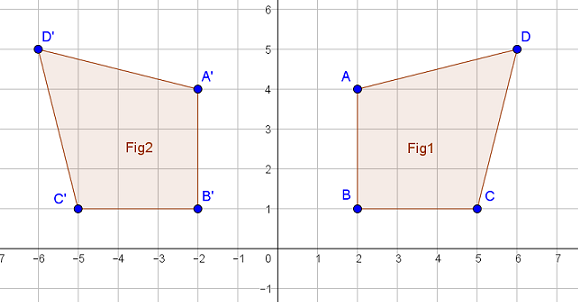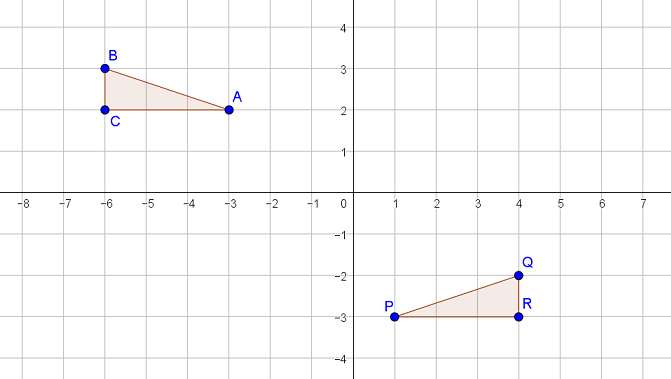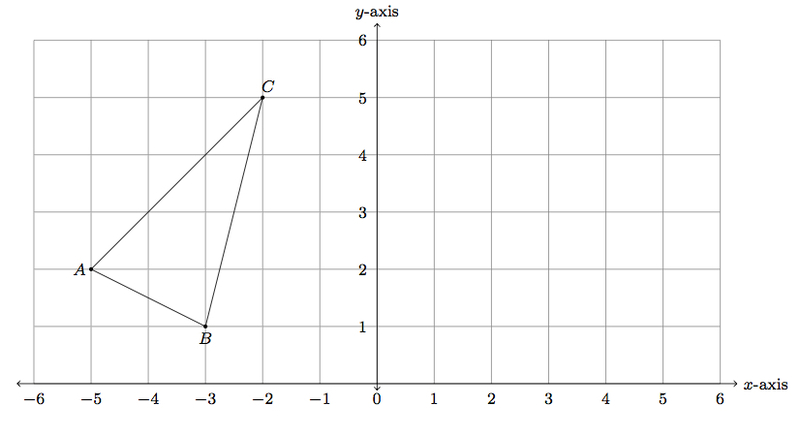# Transformations and Angle Relationships

## Objective

Describe sequences of transformations between figures using reflections and translations. Use coordinate points to represent relationships between reflected figures.

## Common Core Standards

### Core Standards

?

• 8.G.A.1.A — Lines are taken to lines, and line segments to line segments of the same length.

• 8.G.A.1.B — Angles are taken to angles of the same measure.

• 8.G.A.1.C — Parallel lines are taken to parallel lines.

• 8.G.A.2 — Understand that a two-dimensional figure is congruent to another if the second can be obtained from the first by a sequence of rotations, reflections, and translations; given two congruent figures, describe a sequence that exhibits the congruence between them.

• 8.G.A.3 — Describe the effect of dilations, translations, rotations, and reflections on two-dimensional figures using coordinates.

## Criteria for Success

?

1. Perform a combination of transformations, using translations and reflections, on a figure.
2. Describe a sequence of transformations, using translations and reflections, between two figures.
3. Understand that reflecting an image across the $y-$axis results in the $x-$coordinate becoming its opposite value; reflecting an image across the $x-$axis results in the $y-$coordinate becoming its opposite value.

## Tips for Teachers

?

• This is the second lesson on reflections. Students start to think about multiple transformations by combining reflections and translations.
• Students begin reasoning about coordinate points as they connect to transformations, building up to Lesson 9 where they will tackle challenging problems with all types of transformations.
• The following materials are useful for this lesson: patty paper (or transparency paper) and graph paper.

#### Fishtank Plus

• Problem Set
• Student Handout Editor
• Vocabulary Package

## Anchor Problems

?

### Problem 1

Figure 1 is reflected over the $y$-axis to create Figure 2, as shown in the coordinate plane below.1. Describe what you notice about the coordinate points of a figure when it is reflected over the $y$-axis.
2. What do you think happens to coordinate points of a figure when it is reflected over the $x$-axis?

### Problem 2

Triangles ${{ABC}}$ and ${{PQR}}$ are shown below in the coordinate plane.

Describe a sequence of transformations that can map ${{ABC}}$ to ${{PQR}}$## Problem Set

?The following resources include problems and activities aligned to the objective of the lesson that can be used to create your own problem set.

• Examples where students give new coordinate points of figures that are transformed (by a reflection, a translation, or a combination of both)
• Examples where students perform sequences of reflections and translations in the coordinate plane
• Examples where students are given two figures and describe the sequence of transformations (reflections, translations, or combinations); explain why the two figures are congruent, similar to Anchor Problem #2
• Examples of error analysis or incorrectly described sequences

?

Below is a picture of a triangle on a coordinate grid:1. Draw the reflection of ${\triangle ABC}$ over the vertical line through ${x=-2}$. Label the image of $A$ as $A'$, the image of $B$ as $B'$, and the image of $C$ as $C'$.
2. Draw the reflection of $\triangle A'B'C'$ over the vertical line through ${x=2}$. Label the image of $A'$ as $A''$, the image of $B'$ as $B''$, and the image of $C'$ as $C''$.
3. What single rigid transformation of the plan will map ${\triangle ABC}$ to $\triangle A''B''C''$? Explain.

#### References

Illustrative Mathematics Reflecting Reflections

Reflecting Reflections, accessed on Oct. 13, 2017, 4 p.m., is licensed by Illustrative Mathematics under either the CC BY 4.0 or CC BY-NC-SA 4.0. For further information, contact Illustrative Mathematics.

?